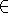Next: Inferring Non-knowledge Up: Logical paradoxesGödel's theorems, Previous: Iterated self-confidence

## Relative consistency

When we cannot prove a theory consistent, we can often show that it is consistent provided some other theory, e.g. Peano arithmetic or ZF is consistent.

In his [Gödel, 1940], Gödel proved that if Gödel-Bernays set theory is consistent, then it remains consistent when the axiom of choice and the continuum hypothesis are added to the axioms. He did this by supposing that set theory has a model, i.e. there is a domain and anpredicate satisfying GB. He then showed that a subset of this domain, the constructible sets, provided a model of set theory in which the axiom of choice and the continuum hypothesis are also true. Paul Cohen proved in 1963 that if set theory has any models it has models in which the axiom of choice and the continuum hypothesis are false.

John McCarthy
Mon Jul 15 13:06:22 PDT 2002